#### Simple if Statement

Simple if is the simplest of all decision making statements. Condition should be in the form of relational or logical expression.

Syntax:

`if <condition>:statements-block1`

In the above syntax if the condition is true statements - block 1 will be executed.

Example

`# Program to check the age and print whether eligible for votingx=int (input("Enter your age :"))if x>=18:print ("You are eligible for voting")`

Output 1:

You are eligible for voting

Output 2:

>>>

As you can see in the second execution no output will be printed, only the Python prompt will be displayed because the program does not check the alternative process when the condition is failed.

Qus. What are operators? What is their function? Give examples of some unary and binary operators.

Ans: “Operators are those symbols used with operands, which tells compiler which operation is to be done on operands.‖ in other words – ―operators are tokens that trigger some computation/action when applied to variables and other objects in an expression.‖

Operators are of following types:

•  Unary operators like (+) Unary Plus, (-) Unary Minus, not etc.
•  Binary Operators like (+) addition, (*) multiplication, and etc.

Qus. What is block/code block/suit in Python?

Ans: Sometimes a group of statements is part of another statement of function. Such a group of one or more statements is called block or code-block or suit in python. e.g.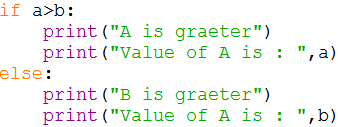Qus. What is the role of indentation in Python?

Ans: Indentation plays a very important role in Python. Python uses indentation to create blocks of code. Statements at same indentation level are part of same block/suit. You cannot unnecessarily indent a statement; python will raise an error for that.

Qus. How many types of strings are supported by Python?

Ans: Python supports two types of strings:

(i) Single-line string That terminates in single line.

(ii) Multi-line String That stores multiple lines of text.

Qus. What will be the sizes of following constants?

(a) "\a‟ (b) “\a” (c) “Kumar\‟s” (d) "\‟ (e) “it‟s”

Ans: (a) 50 (b) 50 (c) 56 (d) 50 (e) 53

This screenshot is the output of the above question.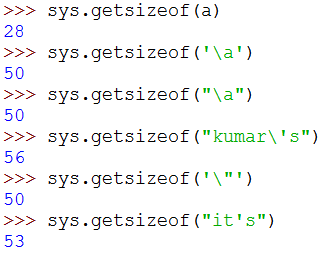Qus. How can you create multi-line strings in Python?

Ans: We can create multi-line string by putting a backslash (\) at the end of line which allows you to continue typing in next line in same string.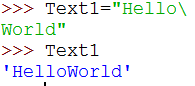Qus. Which of the following are syntactically correct strings? State reason.

(a) ”Python is nice Language”

(b) „He called me “Friend!” when he came‟

(c) “Very Good‟

(d) „This is a good book‟

(e) “Namaste

(f) “I liked „Harry Potter‟ very much”

Ans: (a) Correct (b) Correct (c) Incorrect (d) Correct (e) Incorrect (f) Correct

Qus. What is the error in following Python program with one statement?

print(“My name is : “, name)

suggest a solution

Ans: Error is : ―name 'name' is not defined‖. And the solution is to declare the variable-name before this statement.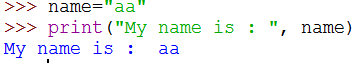Qus. Predict the output of the following: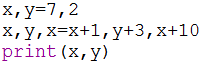Ans: Output: 17 5

Qus. What are augmented assignment operators? How are they useful?

Ans: An augmented assignment is generally used to replace a statement where an operator takes a variable as one of its arguments and then assigns the result back to the same variable. A simple example is x += 1 which is expanded to x = x + (1). Similar constructions are often available for various binary operators. They are helpful in making the source code small.

Qus. What are loops in Python? How many types of loop are there in Python?

Ans: Loops are iteration constructs in Python. Iteration means repetition of a set of statements depending upon a condition test. Loops has three basic elements within it to repeat the statements –

• Initialization (Start)
• Check Condition (Stop)
• Updation (Step)

Python provide two types of loop

(i) Conditional Loop while( (Condition based loop)

(ii) Counting loop for (loop for a given number of times).

Qus. What is the syntax of if-elif statement in Python?

Ans: The syntax of if-elif statement in python is as follows:

If condition1:

#code-block of statements when condition1 is true

elif condion2:

#code-block of statements when condition2 is true

elif condition3:

#code-block of statements when condition3 is true
.

.

.

else:

#code-block of statements when all above conditions are false.

Qus What are jump statements in Python? Name jump statements with example.

Ans: Python offers two jump statements to be used with in loops to jump out of loop-iterations.

These are break and continue statements.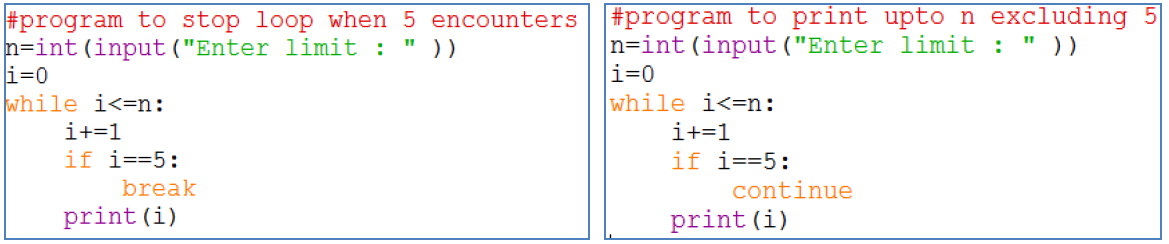#### Programs

python Program to obtain temperature in Celsius and convert it into Fahrenheit

pythoon program to read todays date only date Part from user Then display how many days are left in the current month

python program to print the area of circle when radius of the circle is given by user

python program to print the volume of a cylinder when radius and height of the cylinder is given by user

python program that asks your height in centimeters and converts it into foot and inches

python program accept three side of triangle and find area of a triangle

python program to input principle amount,rate time and calculate simple interes

python program to read a number in n and prints n2 n3 n4

python program to calculate compound simple interest after taking the principle rate and time

python program to take two numbers and check that the first number is fully divisible by second number or not

python program which take value of x y z from the user and calculate the equation

python program to take the temperatures of all 7 days of the week and displays the average temperature of that week

python program to check the given year is leap year or not

python program that searches for prime numbers from 15 through 25

python program to test if given number is prime or not

python program to compute the result when two numbers and one operator is given by user

python program to calculate the roots of a given quadratic equation.

python program to input a digit and print it in words

python program to check whether square root of a given number is prime or not

python program to print first n odd numbers in descending order.

python program to find the average of the list of the numbers entered through keyboard

python program to find the largest number from the list of the numbers entered through keyboard

python program to find the sum of n natural numbers

python program to find the sum of first n even numbers

python program to find the sum of first n odd numbers.

python program to generate a list of elements of Fibonacci Series

python program to find simple interest based upon number of years

python program to input any choice and to implement the following

python program to input any number and to print all natural numbers up to given number

python program to input any number and to find sum of all natural numbers up to given number

python program to input any number and to find reverse of that number

python program to input any string and count number of uppercase and lowercase letters

python program to obtain three numbers and print their sum

Python program to obtain length and breath of a rectangle and calculate its area

python program to calculate body mass index of a person

python program to input a number and print its cube

python program to input two number and swap them

python program to input three number and swap 3 numbers

python program to read 3 numbers in 3variables and swap first two variables with the sums of first and second

python program to input a single digit and print a 3 digit number

python program to accept a integer number and find its sum of digit

python program to count the number of digits in given number

python program to find the factorial value of given number

python program to print factor of given number

python program to check given number is composite number or not

python program to find the largest digit of a given number

python program to find the smallest digit of a given number

python program to print table of entered number

python program to calculate the compound interest

python program to find sale price of an item with given price and discount

python program to calculate the sum of odd numbers divisible by 5 from the range 1 to 100

Python Program to Calculate the Area of a Triangle

python program for addition of two times in hour and minute format

python program for addition of two times in hour minute and second format

python program to check number is positive negative or zero

python program to check number is even or odd

python program to check where is profit or loss

python program to check number is buzz number or not

python program to input assets liabilities and capital then check accounting equation means balanced or not

python program to input total debt and total assets and calculate total debt to total assets ratio and check whether major funded by assets or equity

python program to calculate debt to equity check whether it is risky scenario for investor or not

python program to find the difference between greatest and smallest digits presents in the number

python program to print the frequency of digits present in given number

python program to print Floyds triangle

python program to check whether given number is Armstrong or not

python program to find all prime numbers up to given number

python program to convert decimal number to binary

Python program to convert binary to decimal

python program to print quotient

Python program to calculate simple interest

Python program to calculate area and perimeter of a parallelogram

Python program demonstrating working with power operator

Python program of division operator

Python program of modulus

Python program convert dollars in Rupee

Python program to convert kilometers to miles

Python program to convert the distance in feet to inches yards and miles

Python program to calculate GST Goods and Service Tax

Python credit card program

Python program to print absolute value of number provided by the user

Python program to check divisibility of a number

Python program to print the largest number

Python program to display menu to calculate area of square or rectangle

Python program to print larger number using swap

Python program to calculate electricity bill

Python program to input three number and print in ascending order

Python program to check character is alphabetic character or not

Python program to print letters of word

Python program to print ASCII code for entered message

Python program to print first ten mersenne numbers

python program to obtain x y z from user and calculate expression

python program that reads a number of seconds and prints it in form mins and seconds

python program to take year as input and check if it is a leap year or not

python program to take two numbers and print if the first number is fully divisible by second number or not

python program to take a 2 digit number and then print the reversed number

python program to take a 3 digit number and then print the reversed number

python program to take two inputs for day month and then calculate which day of the year

python program that asks a user for a number of years and then prints out the number of days hours minutes and seconds

python program that inputs an age and print age after 10 years

python program that generates six random numbers in a sequence created with start stop step

python program to generate 3 random integers between 100 and 999 which is divisible by 5

python program to generate 6 digit random secure OTP between 100000 to 999999

python program to generate 6 random numbers and then print their mean median and mode

python program to find a side of a right angled triangle whose two sides and an angle is given

python program to calculate the radius of a sphere whose area is given

python program to calculate the area of an equilateral triangle

python program to input the radius of a sphere and calculate its volume

python program to calculate amount payable after simple interest

python program to input length of three sides of a triangle Then check if these sides will form a triangle or not

python program to input 3 sides of a triangle and print whether it is an equilateral scalene or isosceles triangle

python program to Print numbers from 11 to N When the number is a multiple of 3 print Tipsy when it is a multiple of 7 print Topsy

python program to input N numbers and then print the second largest number

python program to input list of numbers and find those which are palindromes

python program to place and the most significant digit of number

Python program to print every integer between 1 and n divisible by m Also report whether the number that is divisible by m is even or odd

python program to find the LCM of two input numbers

python program to find GCD of 2 number

python program to find the ASCII Value of entered character

python program to check number is perfect number or not

python program to check number is special or not

python program to print first n perfect numbers

Python program using if Elif else statement to find the number of days present in a month

python program to print all buzz numbers between 1 to n

Python program to input 3 numbers and check all are same or not

python program to input 3 numbers from user and check these are unique numbers are not

python program to input 2 integer number and check where they are same of different number